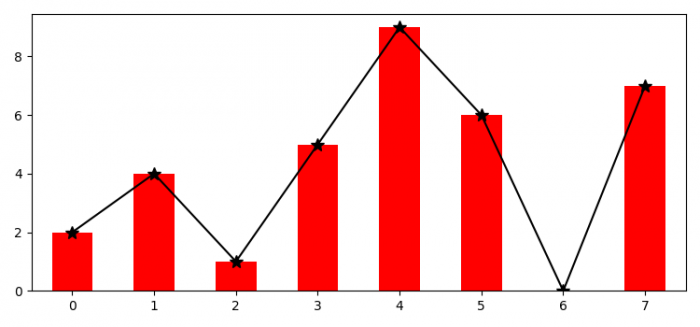# How to show a bar and line graph on the same plot in Matplotlib?

To show a bar and line graph on the same plot in matplotlib, we can take the following steps −

• Set the figure size and adjust the padding between and around the subplots.

• Make a two-dimensional, size-mutable, potentially heterogeneous tabular data.

• Create a figure and a set of subplots.

• Plot the bar and line with the dataframe obtained from Step 2.

• To display the figure, use show() method.

## Example

import pandas as pd
import matplotlib.pyplot as plt

plt.rcParams["figure.figsize"] = [7.50, 3.50]
plt.rcParams["figure.autolayout"] = True

df = pd.DataFrame(dict(data=[2, 4, 1, 5, 9, 6, 0, 7]))
fig, ax = plt.subplots()

df['data'].plot(kind='bar', color='red')
df['data'].plot(kind='line', marker='*', color='black', ms=10)

plt.show()

## OutputUpdated on: 09-Aug-2021

14K+ Views